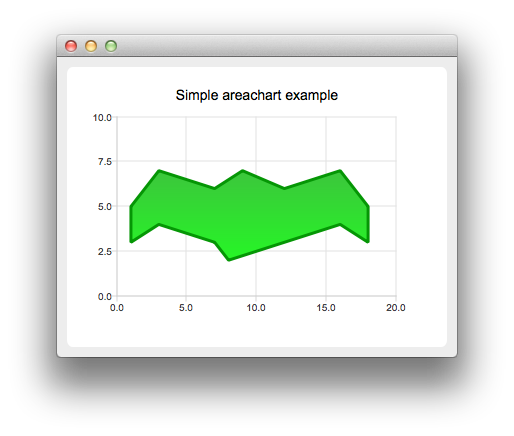# AreaChart Example

The example shows how to create a simple area chart.#### Running the Example

To run the example from Qt Creator, open the Welcome mode and select the example from Examples. For more information, visit Building and Running an Example.

#### Creating Area Charts

To create area charts, we need two QLineSeries instances. They are going to define the upper and lower boundary of the area.

```    QLineSeries *series0 = new QLineSeries();
QLineSeries *series1 = new QLineSeries();```

We add data to both series and use the stream operator.

```    *series0 << QPointF(1, 5) << QPointF(3, 7) << QPointF(7, 6) << QPointF(9, 7) << QPointF(12, 6)
<< QPointF(16, 7) << QPointF(18, 5);
*series1 << QPointF(1, 3) << QPointF(3, 4) << QPointF(7, 3) << QPointF(8, 2) << QPointF(12, 3)
<< QPointF(16, 4) << QPointF(18, 3);```

Now we create a QAreaSeries instance using two line series objects. We set the custom gradient fill and width of the outline.

```    QAreaSeries *series = new QAreaSeries(series0, series1);
series->setName("Batman");
QPen pen(0x059605);
pen.setWidth(3);
series->setPen(pen);

Last we create the QChartView instance, set the title, set anti-aliasing, and add the area series. We also create the default axes and specify the ranges on them.

```    QChart *chart = new QChart();
```    QChartView *chartView = new QChartView(chart);